# Evaluation and Efficiency Measurements of AC Adaptors and Other Products with and without Loads

Application
To evaluate AC adaptors, engineers must acquire the voltage, current, power, and I/O efficiency of input signals (100 VAC) and DC output signals (12 VDC) in both loaded and unloaded states. There are also cases where two outputs come from one AC signal input. The WT500 Power Analyzer handles these tasks efficiently because it can calculate total efficiency and other parameters under those conditions in a single unit.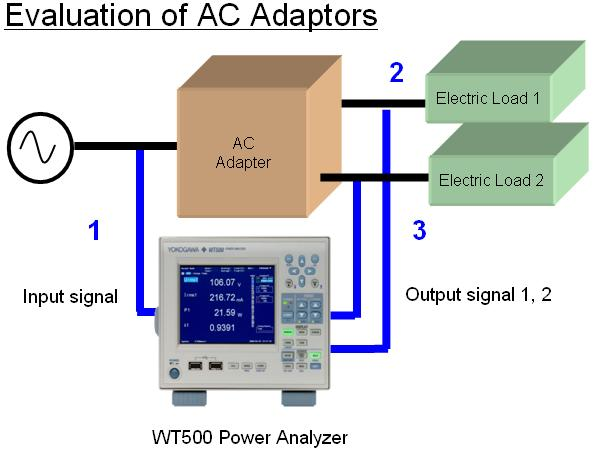You can display the AC voltage, current, and power from the input plus two systems of measured DC voltage, DC current, and power from the output. I/O efficiency ((output power 1 + output power 2) /input power) can also be displayed.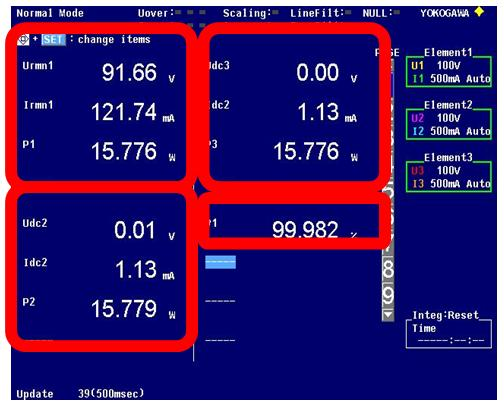* With no load, current can be a repetitive intermittent signal with widely fluctuating current values resulting in unstable power values. In this case, you can average the data by lengthening the data update rate or by using the Average function. If this does not stop the fluctuation, you can calculate the average power value by measuring mean active power with the Integration function.

Calculation of Average Active Power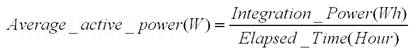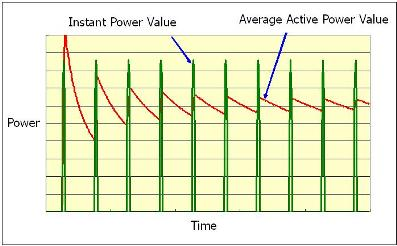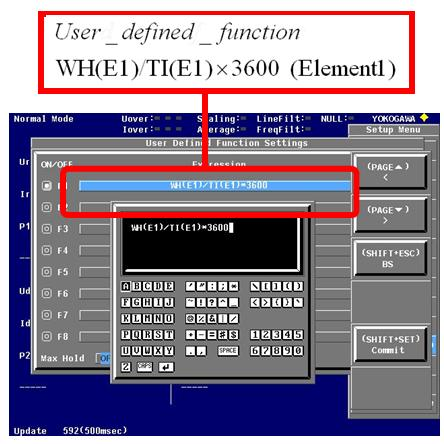Since intermittently-occurring instantaneous power values constantly fluctuate as in the figure above, the displayed value differs at each data update. However, if the repeatedly occurring instantaneous power values are averaged, the average approaches a knowable, constant value that can be confirmed.

Solution Features

1. High accuracy DC and AC measurement
2. Efficiency measurement
3. Simultaneous DC and AC Measurement
4. Average active power calculation
5. Variety of display formats and data saving

WT500 Features

1. AC and DC Accuracy: 0.1% of Reading
2. I/O efficiency ((output power 1 + output power 2) /input power) can be displayed
3. Simultaneous DC and AC Measurement
4. Calculates average power of even unstable signals by measuring mean active power.
5. Measurement values can be saved as images or numerical data, and can be pasted into reports, analyzed in spreadsheet software, or used in a variety of other ways.

### Related Products & Solutions

#### WT500 - Mid Range

• Power and Harmonic (THD) Measurements with Independent Range Controls
• Efficiency measurements of AC/DC or DC/AC Inverters & Motors

Top